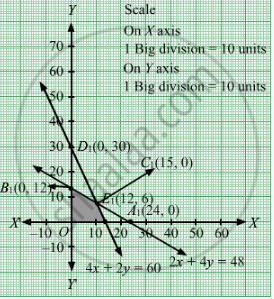# A Company Manufactures Two Articles a and B. There Are Two Departments Through Which These Articles Are Processed: (I) Assembly and (Ii) Finishing Departments. - Mathematics

Sum

A company manufactures two articles A and B. There are two departments through which these articles are processed: (i) assembly and (ii) finishing departments. The maximum capacity of the first department is 60 hours a week and that of other department is 48 hours per week. The product of each unit of article A requires 4 hours in assembly and 2 hours in finishing and that of each unit of B requires 2 hours in assembly and 4 hours in finishing. If the profit is Rs 6 for each unit of A and Rs 8 for each unit of B, find the number of units of A and B to be produced per week in order to have maximum profit.

#### Solution

Let x  units and y units of articles A and B are produced respectively.
Number of articles cannot be negative.
Therefore,  $x, y \geq 0$

The product of each unit of article A requires 4 hours in assembly and that of article B requires 2 hours in assembly and the maximum capacity of the assembly department is 60 hours a week

$4x + 2y \leq 60$

The product of each unit of article A requires 2 hours in finishing and that of article requires 4 hours in assembly and the maximum capacity of the finishing department is 48 hours a week

$2x + 4y \leq 48$

If the profit is Rs 6 for each unit of A and Rs 8 for each unit of B. Therefore, profit gained from​ x units and y units of articles A and B respectively is Rs 6x and Rs 8y respectively.
Total revenue = Z =  $6x + 8y$ which is to be maximised.
Thus, the mathematical formulat​ion of the given linear programmimg problem is

Max Z =  $6x + 8y$

subject to

$2x + 4y \leq 48$
$4x + 2y \leq 60$

$x, y \geq 0$
First we will convert inequations into equations as follows:
2x + 4y = 48, 4x + 2y = 60, x = 0 and y = 0

Region represented by 2x + 4y ≤ 48:
The line 2x + 4y = 48 meets the coordinate axes at A1(24, 0) and $B_1 \left( 0, 12 \right)$  respectively. By joining these points we obtain the line 2x + 4y = 48. Clearly (0,0) satisfies the 2x + 4y = 48. So, the region which contains the origin represents the solution set of the inequation 2x + 4y ≤ 48.
Region represented by 4x + 2y ≤ 60:
The line 4x + 2y = 60 meets the coordinate axes at C1(15, 0) and  $D_1 \left( 0, 30 \right)$ respectively. By joining these points we obtain the line 4x + 2y = 60. Clearly (0,0) satisfies the inequation 4x + 2y ≤ 60. So,the region which contains the origin represents the solution set of the inequation 4x + 2y ≤ 60.

Region represented by ≥ 0 and y ≥ 0:
Since, every point in the first quadrant satisfies these inequations. So, the first quadrant is the region represented by the inequations x ≥ 0, and y ≥ 0.

The feasible region determined by the system of constraints 2x + 4y ≤ 48, 4x + 2y ≤ 60, x ≥ 0 and y ≥ 0 are as follows.T
he corner points are O(0, 0), B1(0, 12), E1(12, 6) and C1(15,0).
The values of Z at these corner points are as follows

 Corner point Z= 6x + 8y O 0 B1 96 E1 120 C1 90

The maximum value of Z is 120 which is attained at E1(12, 6).
Thus, the maximum profit is Rs 120 obtained when 12 units of article A and 6 units of article B were manufactured.

Concept: Graphical Method of Solving Linear Programming Problems
Is there an error in this question or solution?

#### APPEARS IN

RD Sharma Class 12 Maths
Chapter 30 Linear programming
Exercise 30.4 | Q 31 | Page 54